# Mixtures and solutions + system of equations - math problems

#### Number of problems found: 40

• Alcohol solutionsWe have to produce 2 liters of 60% alcohol from 55% and 80%. How many of which ones will we use in the solution?
• Banknotes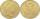\$ 1390 was collected. How much was in \$20 notes and how many in \$50 notes in that order? How many solutions exists?
• SolutionsHow much 60% solution and how much 35% solution is needed to create 100 l of 40% solution?
• Tulips and daffodils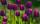Two hundred twenty tulips and daffodils are planted in the flowerbed. One-third of all tulips and one-sixth of all daffodils equals the number of all tulips. How many tulips and how many daffodils?
• Cottages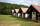The summer camp is 41 cottages. Rooms are for three and for four in them. How many of the 140 campers lives of three?
• Metal alloy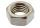What is the ratio of metals in the alloy that is in the 50 tonnes of steel to 30 kg nickel?
• CoinsThe money - coins are minted from the hardest bronze, which contains copper and tin in a ratio of 41: 9. How much copper and tin are in 2kg of bronze money?
• Crown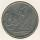Siblings collect a 2-crowns and 5-crowns. Together have 80 coins with a total of value 310 crowns. How many saved two-crowns and how many five-crowns?
• ConcentrationHow many grams of 65% and 50% acid is to be mixed to give 240 g acid with a concentration of 60%?
• Mixing waterThe 30-liter container should we fill with water at 60 degrees Celsius. How many liters of water 80 degrees C hot and how many liters of water 20 degrees Celsius warm we have to mix?
• SolutionWhat amount of 80% solution and what amount of water is needed to mix in order to obtain two liters of 60% solution of this mixture?
• Beds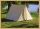At the summer camp, there are 41 chalets. Some rooms are 3-beds, some 4-beds. How many campers from 140 are living in 3-bed?
• BonbonsCreate a mixture of 50 kg of candy on price 700Kč. Candies has prices: 820Kč, 660Kč and 580Kč. Use cross rule.
• Alcohol mixingHow much 55% alcohol we must pour into 1500 g 80% alcohol to form a 60% alcohol? How much 60% alcohol created?
• Water reservoir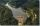The water reservoir is filled through one inlet 4 hours later than both together, then another inlet 9 hours later than both together. For how long is filled by each separately?
• Alcohol mixtureFrom 55% and 80% alcohol we have to produce 0.2 kg of 60% alcohol. How many of them do we use in solution?
• SpiritFrom 55% and 80% spirit we would like to produce 0.2 kg of 60% spirit. How many of them we must use in a solution?
• The pool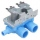The pool contains 220 m3 of water. The pool can be emptied either: a) 10 hours of pipe B and 8 hours of pipe A, or b) 10 hours of pipe A and 7 hours of pipe B. How many cubic meters of water will flow in 1 hour from pipe A and how many from pipe B?
• RingThe ring made from gold and copper alloy has a weight of 14.5 g and a volume of 1.03 cm3. How much gold and how much copper it contains? The metal densities are Au 19.3 g/cm³ and Cu 8.94 kg·dm-3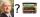Laco went (was sent) to a conference in Amsterdam for a reward. The conference fee was € 2536. Calculate how many books Ladislav could buy at the price of 46 and 54 euros if he doesn't want to fill the whole home library, and he can buy only 52 books